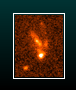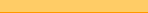GRB hostsProjectDatabasePresentationsPublicationsProposalsRelated linksGlossaryProgramming# XRF 020903

 Redshift: z = 0.2510 RA,Dec: 342.1760 -20.7692 Luminosity distance: D = 1271.00 Mpc Error: 0.00 arcmin Galactic dust extinction: EB-V = 0.03 Duration: T90 = 32.15 s Error: -

## Host

 Stellar mass: log(M*/M☉) = 8.87 Error: 0.07 Metallicity: log(Z/Z☉) = -0.44 Error: - Star formation rate: SFR = 2.65 M☉ yr-1 Error: - Specific star formation: SSFR = - Gyr-1 Error: - Optical extinction: AV = 0.59 Error: -

## Host SED

 Obs. Wavelength (Å) Flux (μJy) References 4,360 8.27  ±   0.76 Bersier et al. (2006) 5,450 17.5   ±   1.6 Bersier et al. (2006) 5,918 14.5   ±   0.7 Wainwright et al. (2007) 6,380 14.5   ±   1.3 Bersier et al. (2006) 7,970 15.4   ±   1.4 Bersier et al. (2006) 2,100,000,000 <  53 Michałowski et al. (2012)

## Host emission line fluxes

 Emission line Flux (10-17 cgs) References [O II] λ3727 39.179 Soderberg et al. (2004) [O II] λ3727 37.6 Levesque et al. (2010) [O II] λ3727 68.9 Hammer et al. (2006) [O II] λ3727 7.982 Bersier et al. (2006) [O II] λ3727 11.48  ±   0.36 Han et al. (2010) [Ne III] λ3869 2.923 Bersier et al. (2006) [Ne III] λ3869 20.5 Hammer et al. (2006) [Ne III] λ3869 9.27 Levesque et al. (2010) [Ne III] λ3869 9.027 Soderberg et al. (2004) [Ne III] λ3970 2.664 Soderberg et al. (2004) [Ne III] λ3970 1.059 Bersier et al. (2006) Hδ 1.272 Bersier et al. (2006) Hδ 3.853 Soderberg et al. (2004) Hδ 5.76 Levesque et al. (2010) Hδ 1.76  ±   0.24 Han et al. (2010) Hγ 3.94  ±   0.29 Han et al. (2010) Hγ 11.99 Levesque et al. (2010) Hγ 7.141 Soderberg et al. (2004) Hγ 2.019 Bersier et al. (2006) [O III] λ4364 5.4 Hammer et al. (2006) [O III] λ4364 0.55  ±   0.07 Han et al. (2010) [O III] λ4364 1.009 Soderberg et al. (2004) Hβ 8.58  ±   0.29 Han et al. (2010) Hβ 44 Hammer et al. (2006) Hβ 3.51 Bersier et al. (2006) Hβ 20.47 Soderberg et al. (2004) Hβ 52.6 Levesque et al. (2010) [O III] λ4959 90.2 Levesque et al. (2010) [O III] λ4959 34.5 Soderberg et al. (2004) [O III] λ4959 7.386 Bersier et al. (2006) [O III] λ4959 74 Hammer et al. (2006) [O III] λ4959 15.56  ±   0.31 Han et al. (2010) [O III] λ5007 44.11  ±   0.33 Han et al. (2010) [O III] λ5007 335 Hammer et al. (2006) [O III] λ5007 20.89 Bersier et al. (2006) [O III] λ5007 113.51 Soderberg et al. (2004) [O III] λ5007 243.7 Levesque et al. (2010) Hα 120 Levesque et al. (2010) Hα 75.01 Soderberg et al. (2004) Hα 11.46 Bersier et al. (2006) Hα 168 Hammer et al. (2006) Hα 26.03  ±   0.21 Han et al. (2010) [N II] λ6585 0.77  ±   0.1 Han et al. (2010) [N II] λ6585 <   0.133 Bersier et al. (2006) [N II] λ6585 1.607 Soderberg et al. (2004) [N II] λ6585 3.16 Levesque et al. (2010) [S II] λ6718 <   3.4 Levesque et al. (2010) [S II] λ6718 23.9 Hammer et al. (2006) [S II] λ6718 2.14  ±   0.17 Han et al. (2010) [S II] λ6718 4.095 Soderberg et al. (2004) [S II] λ6733 2.376 Soderberg et al. (2004) [S II] λ6733 1.28  ±   0.09 Han et al. (2010) [S II] λ6733 17.1 Hammer et al. (2006) [S II] λ6733 <   6.2 Levesque et al. (2010)

## Virtual Observatory resourcesSDSS DR7 image from SkyServer DSS image from STScI

Record created: 11/8/2006 10:56:32 AM, record modified: 1/30/2013 5:25:57 AM